# Basic Takeoff Detailed Process

### Completing the Basic Takeoffs

In virtually any estimate, there will be some takeoffs that will be used multiple times when calculating quantities of specific materials. The estimator should establish what those takeoffs are at the very beginning of the estimating process, record the sums, and then use those totals each time they are needed. For example, if the estimator has calculated the total lineal footage of a specific wall type, then the total could be used in determining a wide range of specific materials quantities such as lineal footage of plate material, number of studs in the wall, square footage of insulation, drywall, quantities of paint and more. This section will go over the process for completing the basic takeoffs for the Arts and Crafts house. The process will begin floor area takeoffs.

#### Floor Area

The square footage of floor area for major portions of the structure are one of the first takeoffs that will need to be calculated. Most real estate transactions identify structures by the square footage of the living space and many of the needed costs of building, such as permits and financing, are calculated using those quantities. These quantities can also be used to calculate material quantities such as floor sheathing and concrete. Examples of these are the square footage of the living area of each floor such as the first floor, the second floor, and the basement. Other floor areas that need to be determined include non-living space such as the garage and porches.

##### Main Floor Living Area

Determining the size of the living area is one of the most fundamental quantities to calculate. The space is traditionally calculated to the outside the exterior walls and, as such, can generally be a fairly simple calculation that can be broken down into simple shapes. Figure 5-6 shows a graphic of the first floor with the main floor area highlighted in light tan and the garage area in gray.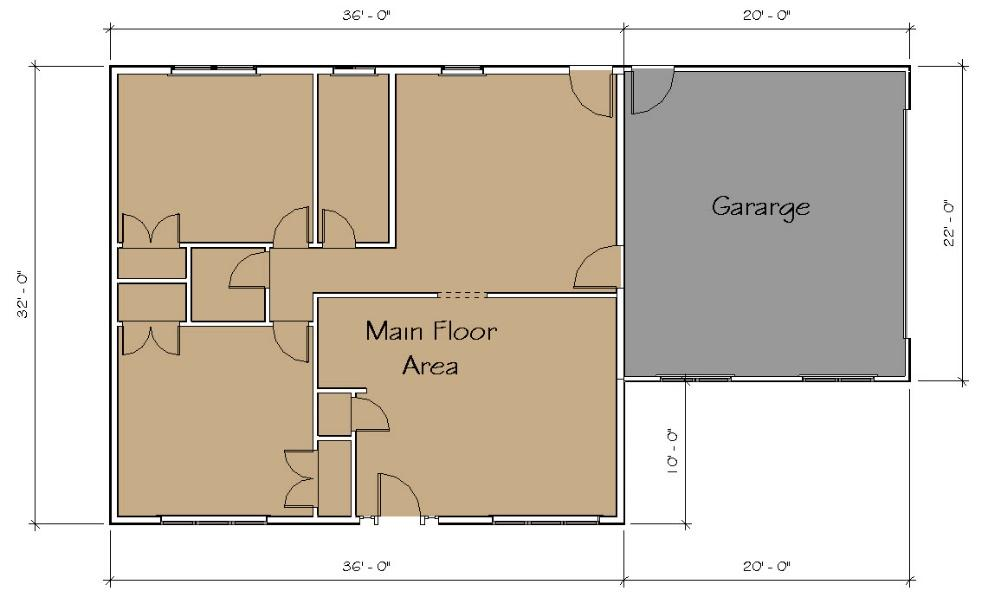Figure 5-6 Graphic representation of the main floor and garage area of the building.

The area in this example is a simple rectangle and can be calculated by multiplying the thirty-six feet length of the rectangle by the thirty-two feet width using the following formula:

$$Length\:\times\:Width\:=\:SF\:Area$$

Or

$$36\:\times\:32\:=\:1,152\:ft^2$$
##### Garage Floor Area

The garage floor area can be calculated in the same fashion. In this case, the area will also be calculated to the exterior of the walls in order to gain an accurate measurement for the area in calculating the building permit. Another approach that would be useful with the garage floor area would be to calculate the area of the concrete floor. In this case, however, only the area to the exterior walls will be calculated as a simple rectangle of 22 feet by 20 feet using the following formula:

$$Length\:\times\:Width\:=\:Area\:ft.^2$$

Or

$$20'\:\times\:22'\:=\:440\:ft.^2$$
##### Basement Floor Area

The basement floor area will be calculated to the interior of the basement walls. This will also include the storage room under the front porch as is shown in Figure 5-7. Three rectangles will be calculated to determine the area. The large main floor rectangle is 34 feet 8 inches long and 35 feet 8 inches wide. The second rectangle under the front porch is 9 feet 8 inches long by 5 feet 7 inches wide. The third rectangle is the small doorway between the two and is 3 feet 2 inches long by 8 inches wide. The following formula will be used to calculate the area:

$$Basement\:Area\:+\:Porch\:Area\:+\:Doorway\:Area\:=\:Total\:Area$$

Or

$$(34^′\mbox{-}8^″\:\times\:30^′\mbox{-}8^″)\:+\:(9^′\mbox{-}8^″\:\times\:5^′\mbox{-}7^″)\:+\:(3^′\mbox{-}2^″\:\times\:8^″)\:=\:Total\:Area$$

Or

$$1063.11\:ft.^2\:+\:53.97\:ft.^2\:+\:2.11\:ft.^2\:=\:1119.19\:ft.^2$$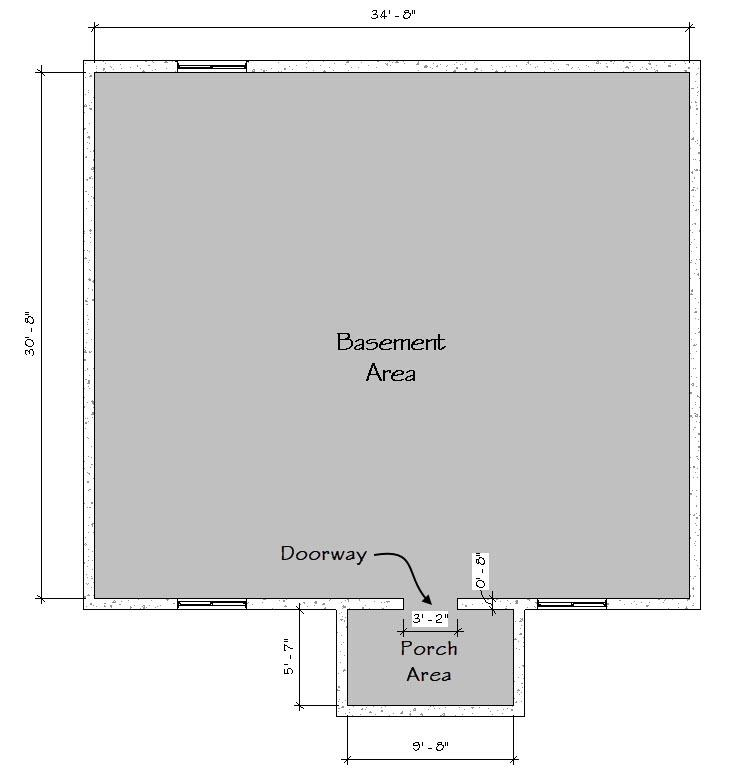Figure 5-7 The basement floor area square footage is comprised of three separate floor areas.

#### Completing the Footings and Foundation Takeoffs

The next basic takeoffs to be completed will be the footing and foundation sections. Both sections show two distinct styles of footing and foundation. The two footing styles are the exterior wall footings and the interior grade footing. The two foundation styles are the main foundation and the garage foundation. This is shown in Figure 5-8.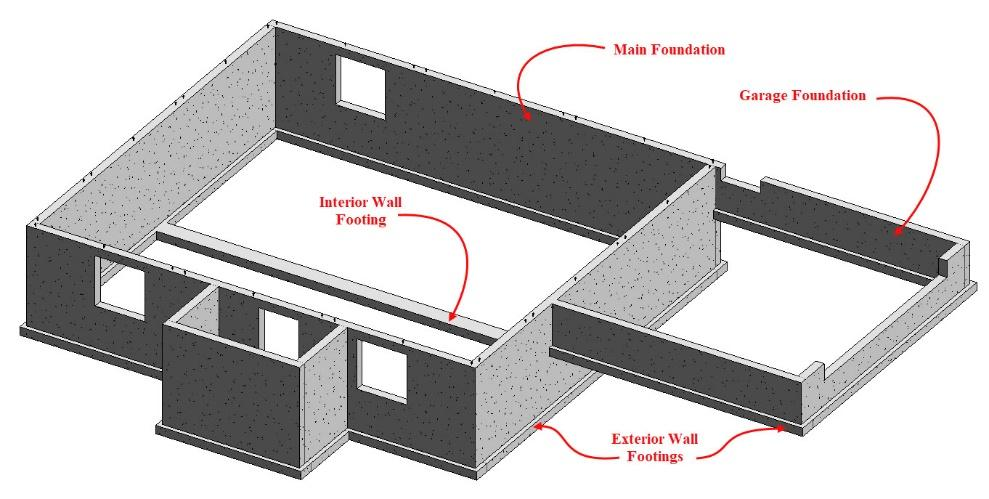Figure 5-8 Three-dimensional footing and foundation view showing different footing and foundation styles.
##### Footing Takeoffs

The footings below both the main foundation and garage foundation are classified as exterior wall footings even though they support different foundation types. This is because both footings are identical in size, shape, and construction. The footings are 16 inches wide and 8 inches deep. Each footing also has two pieces of continuous horizontal rebar and vertical rebar dowel spaced at 32 inches on-center. The interior wall footing is also 16 inches wide by 8 inches deep and also has two pieces of continuous horizontal rebar, however, these footings do not have any vertical rebar dowel and, as such, are defined as a different style. This is shown in Figure 5-9.

The particulars of footing and foundation size and construction information can usually be determined from section view drawings such as full building sections or more detailed wall sections. Figure 5-10 shows an example of a detailed footing and foundation wall section. The detail shows both dimensions and annotations listing the footing and foundation sizes and annotations describing the location, size, and spacing of the reinforcing rebar.

The length of the footings and foundations should be calculated in such a manner that corners are only counted once. If the measurements are calculated using only the exterior dimensions of the footing or foundation, the corners will be double counted resulting in excess concrete. If the measurements are calculated using only the interior dimensions, the corner will not be counted resulting in a shortage of concrete. One of several strategies could be employed to properly count the length of the footing or foundation.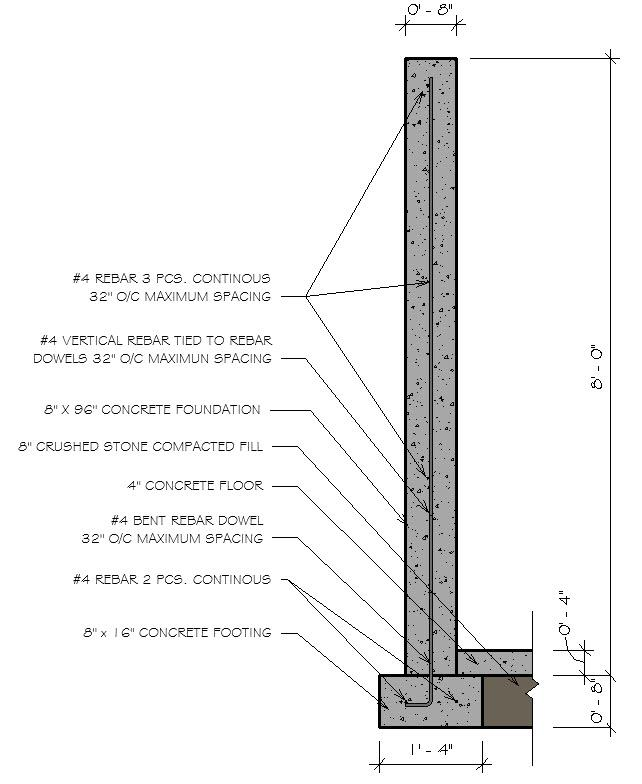Figure 5-10 Footing and foundation wall section detailing the construction specifics.

Three possible approaches would be, (1) measure both the exterior dimensions and interior dimensions of the footings. Add both dimensions together and divide in half to find the average length. (2) Calculate the total length by measuring from the center of the footing. (3) Break the footings into footings that are placed on the page horizontally and vertically. Calculate the horizontal footing by measuring to the outside of the footing and the vertical footings by measuring to the inside of the footings. This is the approach that will be used in the following example.

Figure 5-11 shows a dimensioned plan view of the footings. The footings which are placed horizontally on the page are dimensioned to the outside of the wall and are highlighted in yellow. The footings placed vertically on the page are dimensioned to the inside of the wall and are highlighted in green. The horizontal garage footings are measured to the basement foundation.

##### Foundation Takeoffs

The foundation takeoffs would be completed in similar fashion. The horizontal foundation elements would be dimensioned to the exterior of the wall and the vertical foundation elements would be dimensioned to the inside of the wall. The three-dimensional footing and foundation graphic shown in Figure 5-8 shows two separate foundation types: a main foundation and a garage foundation. These two types have different dimensions and reinforcing requirements and, as such, are treated as separate takeoffs. Figure 5-12 shows a dimensioned plan view of the foundation. The foundations which are placed horizontally on the page are dimensioned to the outside of the walls and are highlighted in dark blue. The foundations placed vertically on the page are dimensioned to the inside of the wall and are highlighted in light blue as shown in Figure 5-12. The garage foundation is a separate takeoff and is not highlighted in this graphic.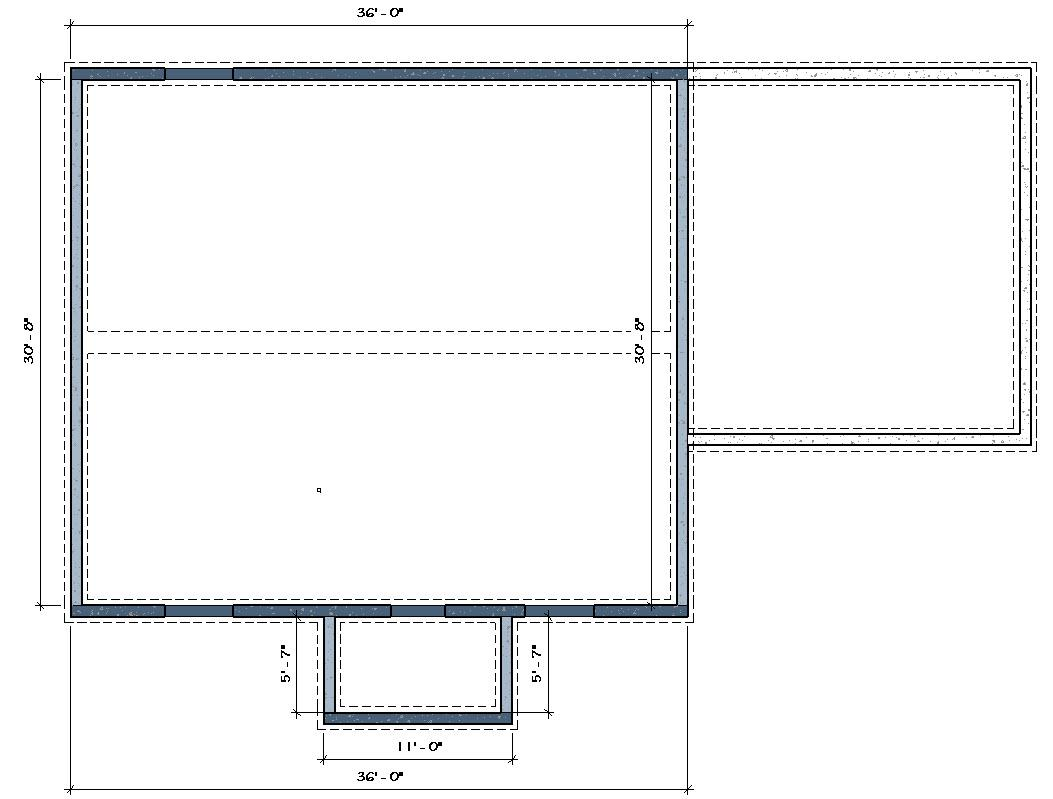Figure 5-12 Graphic showing the lineal footage of a foundation floor plan.

#### Completing the Wall Takeoffs

The wall takeoffs will be completed in similar fashion as the footing and foundation takeoffs. There are a number of different wall types for both exterior and interior walls. There are also a number of different ways of classifying the walls. For the purpose of this exercise, walls will be classified primarily by their framing structure such as stud size, including width, length, and spacing. The plate layout includes the number of top and bottom plates. The wall sheathing that is installed during the framing phase. In most cases, wall finishes will not be taken into account in classifying different wall types. For example, exterior walls may have a brick or stone veneer finish, they may have a wood, or vinyl siding, or the exterior finish may be a combination of several finish types. If the structure of the framing is the same, the walls will be classified as the same type. The wall takeoffs will be divided into three broad categories of takeoffs. Each category will be estimated separately during the framing portion of the estimate. The three categories are basement and pony walls, exterior walls, and interior walls.

##### Basement and Pony Wall Takeoffs

The basement and pony walls are walls that are framed in the basement or on the foundation to support the floor system. In addition, they are also walls framed around the inside of the foundation to provide a structure for installing insulation and wall finishes. In addition, walls to frame out the various basement rooms are included. Figure 5-13 shows the walls in the basement. In this example, there are two wall types basement bearing walls that are used to support the floor. These walls have a double top plate and are framed using 2 × 4’s spaced 16 inches on-center. The other wall type is an insulation wall. These walls are framed to provide a place to install insulation. They are framed using a single top plate with a stud spacing of 24 inches on-center.

The actual construction of each wall type can best be determined by referencing building sections or wall section detail drawings. Most often, notes and annotations will detail the wall construction similar to what was previously shown when calculating the footing and foundation walls. Figure 5-14 shows an expanded section view of the footing and foundation wall section shown in Figure 5-10. The view shows the addition of the basement insulation wall. Bolded annotations in this graphic specifically detail the construction of this wall type. Of particular importance in this instance is the size of the studs, the size, type and number of the plates, and the stud spacing.

The corners of wood framed walls should also be counted only a single time as was done for the footing and foundation walls to avoid any overlap. At this early stage of the estimating process, the quantities calculated should be as precise as possible and contain only the raw numbers. Other factors such as waste factors or contingencies can be added later in the estimating process.

Figure 5-15 shows a floor plan view of the basement walls with the insulation walls highlighted in pink and the bearing walls highlighted in blue along with dimensions needed for calculating the lineal footage of both wall types.

Determining the actual wall length to enter often takes a little bit of interpretation of the drawings. The outside dimensions of the walls are measured from the exterior of the building, or, in this case, the inside of the basement foundation. The interior measurements are dimensioned to the center of the wall. This conforms to standard construction methods where the carpenter lays out the wall to the center and then marks the offset from the center to the exterior of one side when installing the walls. Because of this wall placement, will be dimensioned in the drawings in fractions of an inch. The fractions can usually be ignored and either truncated or rounded up to the next whole number during the takeoffs. Figure 5-42 shows the bearing walls entered into the spreadsheet calculator with the horizontal walls entered first and the vertical walls second. Doors and openings less than 16 feet are ignored in completing the takeoff. The door in the center bearing walls just to the right of the stairway is ignored in this case and the wall calculated the entire length as shown.

The insulation walls would be determined in similar fashion.

##### Exterior and Interior Wall Takeoffs

Three wall types are included in the exterior wall category in the basic takeoffs. The three wall types are 2 × 6 exterior walls, 2 × 6 common walls, and 2 × 4 garage walls. Figure 5-16 shows a close-up view of the front corner of the building where the three exterior wall types intersect. The 2 × 6 exterior walls, and the 2 × 6 common walls are built on the floor structure and, technically, would be framed as a single wall, however, for the purpose of calculating the basic takeoffs, they will be separated into the two types because they are constructed differently. The exterior wall is sheathed with OSB sheathing and the common wall is covered with drywall on both sides. The 2 × 4 garage walls are displayed in blue and are built on the foundation walls. These walls have a different construction in that studs are 2 × 4’s as opposed to 2 × 6’s in the other types. The studs are also longer because they go all of the way to the foundation instead of resting on the floor system. In addition, the bottom plate is pressure treated material.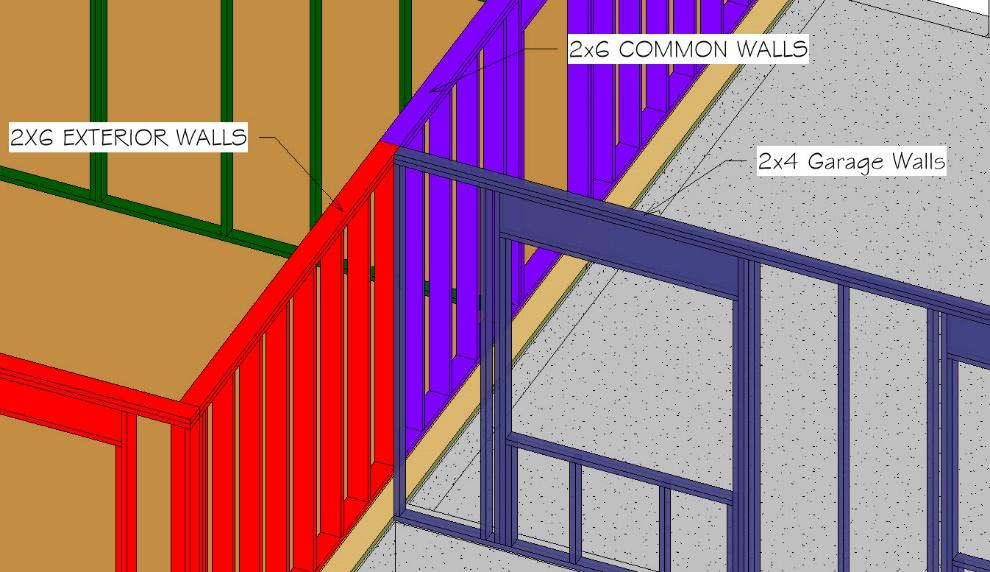Figure 5-16 Exterior corner showing the intersection of three exterior wall types.

Figure 5-17 shows a color-coded graphic of all of the main floor walls, including the exterior walls and interior walls. Calculating the total length of these wall types is done in a manner similar to what has been previously done. Each wall type is identified using the floor plans and section drawings taking care to not double count the corners.

#### Completing the Roof Takeoffs

The roof subsection of the basic takeoffs is comprised of three quantities which are, square footage of flat roof area, lineal feet of roof ridge, and lineal feet of roof edge.

##### Square Footage of Flat Roof Area

The square footage of the flat roof area is calculated as if it were a flat roof. This number includes all of the roof area including overhangs. This number can be used later in the estimating process to calculate the sloped roof area. Figure 5-18 shows a dimensioned roof plan. The roof plan does not show a small area of the garage roof that is under the main roof. This roof area is equal to the distance of the roof overhang multiplied by half of the roof width. This is highlighted by the area outlined in red.

##### Lineal Footage of Roof Ridge Line

The roof plan in Figure 5-18 can also be used to determine the lineal footage of the roof ridge line. Three ridge lines are shown; the main roof ridgeline, the garage roof ridgeline, and the front porch ridgeline. The length of both the main roof and garage ridge lines can be determined from the dimensions. To determine the length of the front porch ridgeline, add the 5′-10 ¼″ roof edge distance to the roof run to find the total length. In this case, the span of the roof is 12′-1 ½″ and the run would be half of that distance, or 6′-0 ¾″. The total length of the front porch ridgeline would be

$$5^′\mbox{-}10\:\tfrac{1}{4}^″\:+\:6^′\mbox{-}0\:\tfrac{3}{4}^″\:=\:11^′\mbox{-}11^″$$
##### Lineal Footage of Roof Edge

The lineal footage of roof edge is a little more complicated than the other two roof basic takeoffs. This takeoff follows the edge of the roof around the building. It includes both the horizontal roof edges and sloped roof edges. Figure 5-19 shows the east side elevation of the house and highlights in red the roof edges.

This view shows both horizontal roof edges and sloped roof edges. The single horizontal roof can be determined from the 6′-3′ dimension. The sloped roof edges will need to be calculated. The roof slope is shown as 6:12. The slope length of the roof is a triangle that is half of the roof span. Half of the roof span is commonly called the roof run. Two spans are given. The smaller garage span of 22 feet is listed. The house span is determined by calculating the garage and the additional width of the house and is 32 feet. Half of each span, or the run, would be as follows:

$$22^′\mbox{-}0^″\:\div\:2\:=\:11^′\mbox{-}0^″\:Run$$
$$32^′\mbox{-}0^″\:\div\:2\:=\:16^′\mbox{-}0^″\:Run$$

The total roof edge on this side of the house would be equal to the sloped roof edges of the house multiplied by two and one garage sloped roof edge. In addition, the horizontal run of the side of the front porch would be added. The run and the roof slope ratio can be used to calculate the vertical roof rise by multiplying the run by the slope ratio. The garage roof rise can be calculated by doing the following:

$$\tfrac{6}{12}\:Ratio\:\times\:11^′\mbox{-}0^″\:Run$$

Or

$$\tfrac{1}{2}\:\times\:11^′\mbox{-}0^″\:=\:5^′\mbox{-}6^″\:Rise$$

The main house roof rise can be calculated by doing the following:

$$\tfrac{6}{12}\:Ratio\:\times\:16^′\mbox{-}0^″\:Run$$

Or

$$\tfrac{1}{2}\:\times\:16^′\mbox{-}0^″\:–\:8^′\mbox{-}0^″\:Rise$$

The three sloped lengths are added to the horizontal length to calculate the total length of the roof edge on this side of the house. The total would be as follows:

$$17.89^′\:+\:17.89^′\:+\:12.3^′\:+\:6.25^′\:=\:54.33^′$$

This total would be added to the totals for the other side of the roof to find the total length of roof edge.

#### Completing the Window and Door Basic Takeoffs

The majority of information to complete both the window and door takeoffs can be obtained from the door and window schedules. Copy the information into the appropriate section in the basic takeoffs. Figure 5-20 shows an example of the window schedule and the information entered into the basic takeoffs is shown in Figure 5-21.